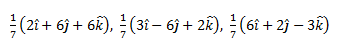Checkout JEE MAINS 2022 Question Paper Analysis : Checkout JEE MAINS 2022 Question Paper Analysis :

# Important Questions Class 12 Maths Chapter 10 - Vectors

Important Questions For Class 12 Maths Chapter 10 Vector Algebra are given here to help the students with their CBSE board exam preparation. These questions will provide you with a clear idea of what type of questions will be asked from the syllabus in the examination. All the related concepts of Vectors are provided with suitable examples at BYJU’S.

All the chapters of Class 12 have different weightage for the CBSE exam along with varying levels of difficulty with respect to the questions of NCERT textbook as well as concepts. Students can refer to the important questions for all the chapter of Class 12 Maths as per the new exam pattern.

## Important Questions & Answers For Class 12 Maths Chapter 10 Vector Algebra

Q. No. 1: Represent graphically a displacement of 40 km, 30° east of north.

Solution: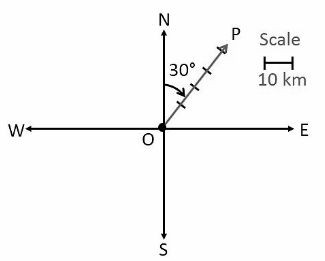Hence, the vector

$$\begin{array}{l}\vec{OP}\end{array}$$
represents the displacements of 40 km, 30° east of north.

Q. No. 2: Find the unit vector in the direction of the sum of the vectors

$$\begin{array}{l}\vec{a}=2\hat{i}-\hat{j}+2\hat{k}\end{array}$$
and
$$\begin{array}{l}\vec{b}=-\hat{i}+\hat{j}+3\hat{k}\end{array}$$
.

Solution:

Let

$$\begin{array}{l}\vec{c}\end{array}$$
be the sum of
$$\begin{array}{l}\vec{a}\end{array}$$
and
$$\begin{array}{l}\vec{b}\end{array}$$
.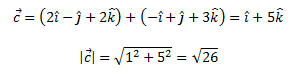The unit vector is: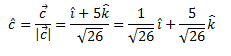Q. No. 3: Find the vector joining the points P(2, 3, 0) and Q(– 1, – 2, – 4) directed from P to Q.

Solution:

Since the vector is to be directed from P to Q, clearly P is the initial point and Q is the terminal point.

P(2, 3, 0) = (x1, y1, z1)

Q(-1, -2, -4) = (x2, y2, z2)

Vector joining the points P and Q is: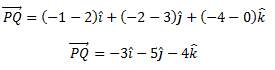Q. No. 4: Find a vector in the direction of a vector

$$\begin{array}{l}5\hat{i}-\hat{j}+2\hat{k}\end{array}$$

which has a magnitude of 8 units.

Solution:

Let

$$\begin{array}{l}\vec{a}=5\hat{i}-\hat{j}+2\hat{k}\end{array}$$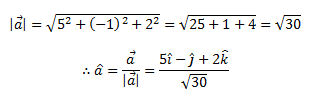Hence, the vector in the direction of vector

$$\begin{array}{l}5\hat{i}-\hat{j}+2\hat{k}\end{array}$$
which has a magnitude of 8 units is given by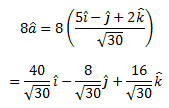Q. No. 5: Show that the vector

$$\begin{array}{l}\hat{i}+\hat{j}+\hat{k}\end{array}$$
is equally inclined to the axes OX, OY and OZ.

Solution:

Let

$$\begin{array}{l}\vec{a}=\hat{i}+\hat{j}+\hat{k}\end{array}$$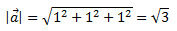Therefore, the direction cosines of

$$\begin{array}{l}\vec{a}\end{array}$$
are (1/√3, 1/√3, 1/√3).

Let α, β, γ be the angles formed by

$$\begin{array}{l}\vec{a}\end{array}$$
with the positive directions of x, y, and z-axes.

Then,

cos α = 1/√3, cos β = 1/√3 cos γ = 1/√3

Hence, the given vector is equally inclined to axes OX, OY and OZ.

Q. No. 6: Show that the points A, B and C with position vectors

$$\begin{array}{l}\vec{a}=3\hat{i}-4\hat{j}-4\hat{k},\ \vec{b}=2\hat{i}-\hat{j}+\hat{k}\end{array}$$
and
$$\begin{array}{l}\vec{c}=\hat{i}-3\hat{j}-5\hat{k}\end{array}$$
form the vertices of a right-angled triangle.

Solution:

Position vectors of points A, B and C are respectively given as below.

$$\begin{array}{l}\vec{a}=3\hat{i}-4\hat{j}-4\hat{k},\ \vec{b}=2\hat{i}-\hat{j}+\hat{k}\end{array}$$
and
$$\begin{array}{l}\vec{c}=\hat{i}-3\hat{j}-5\hat{k}\end{array}$$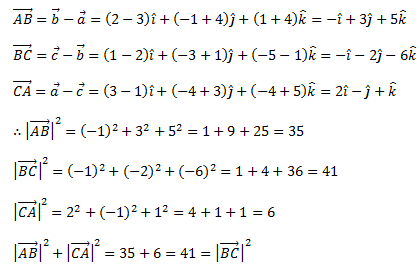Therefore, ABC is a right-angled triangle.

Q. No. 7: Find a vector

$$\begin{array}{l}\vec{r}\end{array}$$
of magnitude 3√2 units which makes an angle of π/4 π/2 with y and z-axes, respectively.

Solution:

From the give,

m = cos π/4 = 1/√2

n = cos π/2 = 0

Therefore, l2 + m2 + n2 = 1

l2 + (½) + 0 = 1

l2 = 1 – ½

l = ±1/√2

Hence, the required vector is: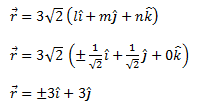Q. No. 8: Evaluate the product.

$$\begin{array}{l}(3\vec{a}-5\vec{b}).(2\vec{a}+7\vec{b})\end{array}$$

Solution: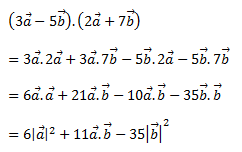Q. No. 9: Find all vectors of magnitude 10√3 that are perpendicular to the plane of

$$\begin{array}{l}\hat{i}+2\hat{j}+\hat{k}\end{array}$$
and
$$\begin{array}{l}-\hat{i}+3\hat{j}+4\hat{k}\end{array}$$
.

Solution:

Let

$$\begin{array}{l}\vec{a}=\hat{i}+2\hat{j}+\hat{k}\\ \vec{b}=-\hat{i}+3\hat{j}+4\hat{k}\end{array}$$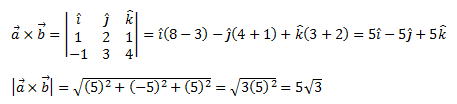Hence, the unit vector perpendicular to the plane of

$$\begin{array}{l}\vec{a}\end{array}$$
and
$$\begin{array}{l}\vec{b}\end{array}$$
is: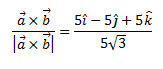Therefore, the vectors of magnitude 10√3 that are perpendicular to the plane of

$$\begin{array}{l}\vec{a}\end{array}$$
and
$$\begin{array}{l}\vec{b}\end{array}$$
are: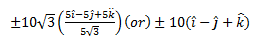Q. No. 10: Find the area of the triangle with vertices A(1, 1, 2), B(2, 3, 5) and C(1, 5, 5).

Solution:

Vertices of a triangle ABC are A(1, 1, 2), B(2, 3, 5) and C(1, 5, 5).

Let AB and BC be the adjacent sides of triangle ABC.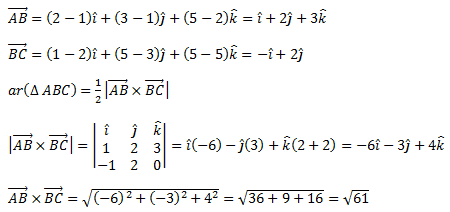Hence, the area of triangle ABC is √61/2 sq.units

### Practice Questions For Class 12 Maths Chapter 10 Vector Algebra

1. Using vectors find the area of triangle PQR, whose vertices are P(1, 2, 3), Q(2, -1, 4) and C(4, 5, -1).
2. Use vector method to show that P,Q,R are Collinear – P(3, -5, 1), Q(-1, 0, 8) and C(7, -10, -6)
3. Find the angles between the lines whose direction ratios are 3, 2, -6 and 1, 2, 2. Find the angles of a triangle PQR whose vertices are P(-1, 3, 2), B(2, 3, 5) and C(3, 5, -2).
4. Prove that the points P(-2, 4, 7), Q (3, -6, -8) and R(1,-2,-2) are collinear.
5. Find the angle between the lines whose direction ratios are: 2, -3, 4 and 1, 2, 1.
6. Using vectors, find the value of k such that the points (k, – 10, 3), (1, –1, 3) and (3, 5, 3) are collinear.
7. If A, B, C, D are the points with position vectors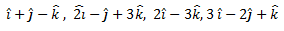respectively, find the projection of
$$\begin{array}{l}\vec{AB}\end{array}$$
along
$$\begin{array}{l}\vec{CD}\end{array}$$
.
8. Using vectors, prove that the parallelogram on the same base and between the same parallels are equal in area.
9. Show that: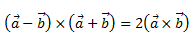10. Show that each of the given three vectors is a unit vector: